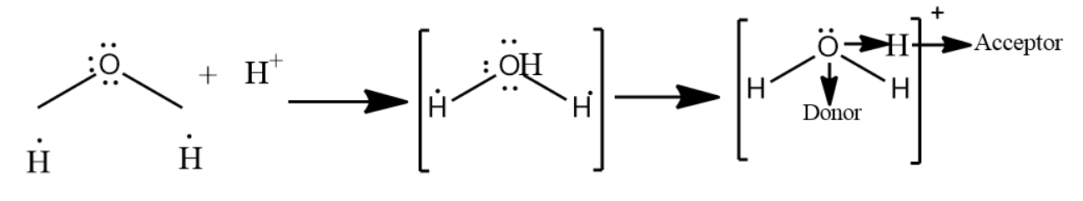QuestionAnswers

# Which combination is best explained by the coordinate covalent bond?(A)- ${{H}_{2}}+{{I}_{2}}$ (B)- $Mg+\frac{1}{2}{{O}_{2}}$ (C)- Cl + Cl(D)- ${{H}^{+}}+{{H}_{2}}O$

Hint: A bond formed by sharing a pair of electrons by two atoms from one atom only is called a coordinate bond or a dative covalent bond. Hydronium ions have two O-H covalent bonds and one O-H coordinate bond which after the formation of $O\to {{H}^{+}}$ co-ordinate bond becomes identical to the two O-H covalent bonds. Hence in ${{H}_{3}}{{O}^{+}}$ ion all the three bonds are identical.

-A covalent bond is a kind of 2-center, 2-electron bond in which the two electrons derived are from the same atom.
-This type of bonding is seen in coordination compounds where a metal ion is bonded to ligands.

- In the first option, the combination given is ${{H}_{2}}+{{I}_{2}}$ which forms 2HI. The HI molecule exists with a covalent molecule with H and I sharing one electron each.

-In the second option, the combination given is $Mg+\frac{1}{2}{{O}_{2}}$ which forms MgO. In MgO molecules, oxygen gains two electrons to complete its octet and the bond formed is an ionic bond.

-In the third option, the combination given is Cl + Cl which forms a chlorine molecule. In $C{{l}_{2}}$ , both atoms share and tightly hold each other’s electrons and form a covalent bond.
-In the fourth option, the combination given is ${{H}^{+}}+{{H}_{2}}O$ which forms ${{H}_{3}}{{O}^{+}}$ . The bonding in the hydronium ion is a coolant bond, in which one electron comes from the oxygen and one comes from the hydrogen.Therefore, the best combination explaining the coordinate bond is the combination of ${{H}^{+}}+{{H}_{2}}O$
So, the correct answer is “Option D”.

Note: Hydronium is the common name of aqueous cation which is a type of oxonium ion produced by protonation of water. Hydronium ion has a trigonal pyramidal molecular geometry with the oxygen atom at its apex. The H-O-H bond angle is approximate $113{}^\circ$ .